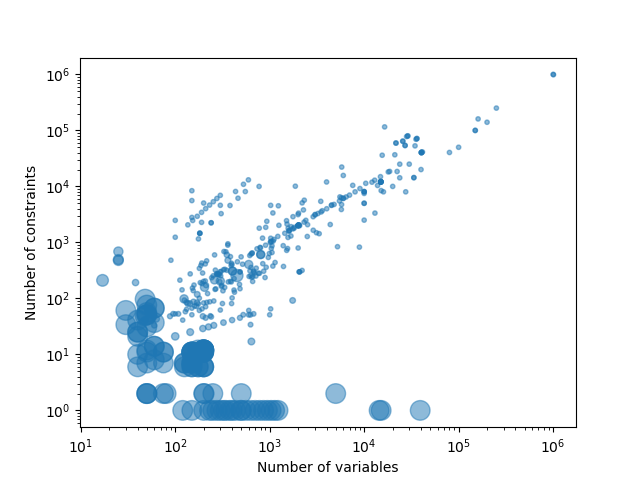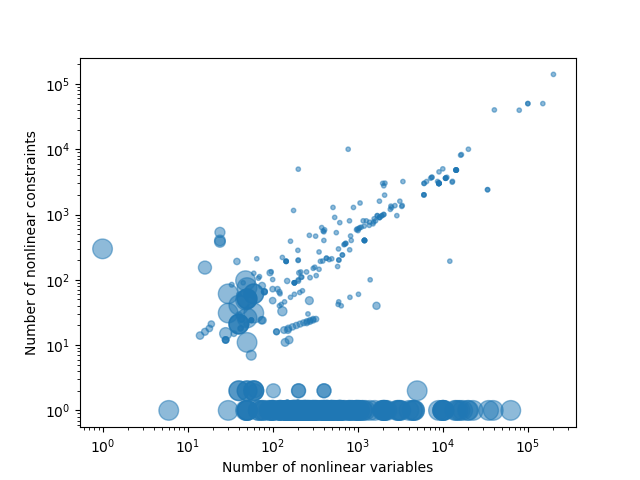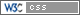# QPLIB

## A Library of Quadratic Programming Instances

Home // browse instances // view statistics // read documentation // download QPLIB archive [ 1.5G ]

The following diagrams provide aggregated statistics on sources and characteristics of the instances in QPLIB. You can download the raw data in csv format. The data fields are defined in the documentation.

### Problem types

For a definition of the three letter problem classification see the documentation.

#### Discrete Instances

 CCB 3 16 11 13 52 3 6 30 134 32 102
 CBL 5 12 6 9 3 2 14 134 91 23 5 1 2 11 1 319 31 288

### Convexity

Left: Convexity of the continuous relaxation. Right: Fraction of problematic eigenvalues in the coefficient matrix of the objective function w.r.t. the total number of variables.

### Problem sizes

See also the definition of nonzeros and nonlinear nonzeros in the documentation.

### Density

In these scatter plots, each bubble represents an instance and the area of the bubble corresponds to the (nonlinear) density of the instance:The diagram below shows the instances with a quadratic objective function sorted according to the density of the quadratic objective function coefficient matrix.